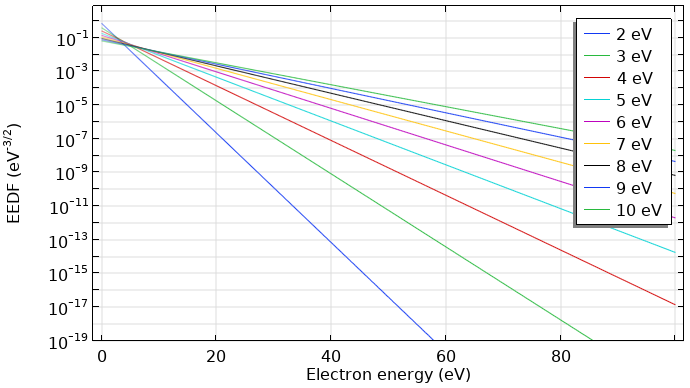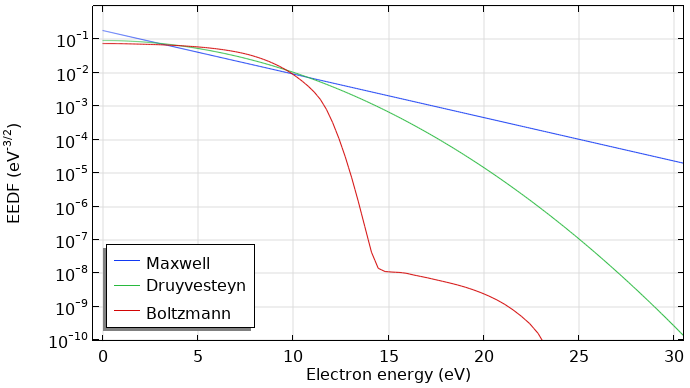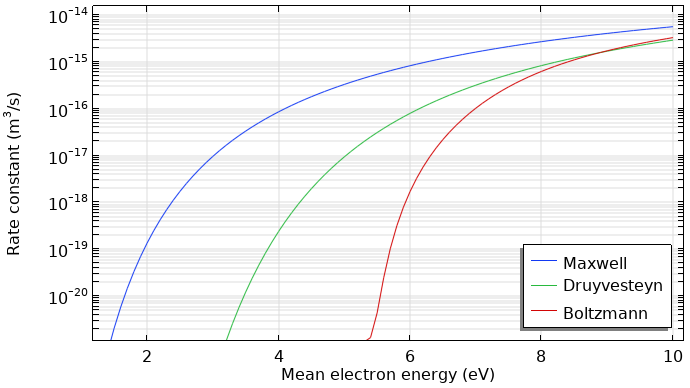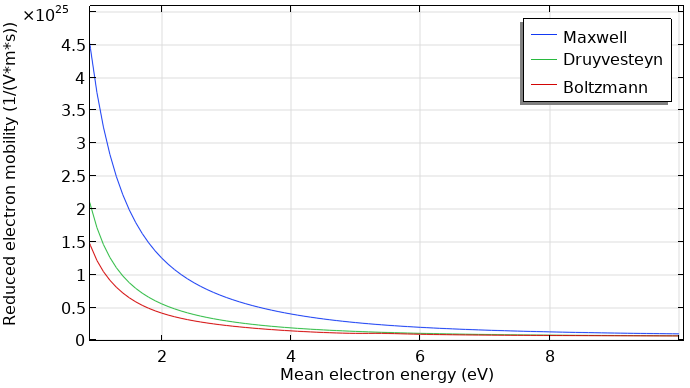# 电子能量分布函数

2014年 8月 4日

### 描述电子能量分布函数

#### 用于描述电子能量分布的函数

 麦克斯韦函数 f(\epsilon)=\varphi^{-3/2}\beta_1\exp\left(-\frac{\epsilon\beta_2}{\varphi}\right) \beta_1=\Gamma(5/2)^{3/2}\Gamma(3/2)^{-5/2},\ \beta_2=\Gamma(5/2)\Gamma(3/2)^{-1} Druyvesteyn 函数 f(\epsilon)=\varphi^{-3/2}\beta_1\exp\left(-\left(\frac{\epsilon\beta_2}{\varphi}\right)^2\right) \beta_1=\Gamma(5/4)^{3/2}\Gamma(3/4)^{-5/2},\ \beta_2=\Gamma(5/4)\Gamma(3/4)^{-1} 广义函数 f(\epsilon)=\varphi^{-3/2}\beta_1\exp\left(-\left(\frac{\epsilon\beta_2}{\varphi}\right)^g\right) \beta_1=\Gamma(5/2g)^{3/2}\Gamma(3/2g)^{-5/2},\ \beta_2=\Gamma(5/2g)\Gamma(3/2g)^{-1}

#### 麦克斯韦函数#### 玻尔兹曼方程

\frac
{\partial f} {\partial t}
+\mathbf
{v}\cdot\triangledown f-\frac{e}{m}(\mathbf{E}\cdot\triangledown_\mathbf{v}
f)=C[f]

### 麦克斯韦、Druyvesteyn 函数与计算的分布函数的比较### 速率系数和传递参数

k_k=\gamma\int\limits_{0}^{\infty}
\epsilon\sigma_k(\epsilon)f(\epsilon)\mathrm d\epsilon